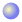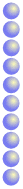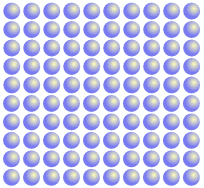# Place Value

We write numbers using only ten symbols (called Digits).
Where we place them is important.

## The Ten Digits

The Digits we use today are called "Hindu-Arabic Numerals":

0   1   2   3   4   5   6   7   8   9

We can use these on their own to count up to 9:

 0 123456789??But what happens after 9?

## Ten Or More ...

When we have more than 9 items, we start another column - the "tens" column - and we write down how many "tens" we have, followed by how many "ones" (also called "units").

So we write:

 10### Example: this is how we write down twelve:

 Tens Ones 1 2The Number "12"It says we have 1 Ten and 2 Ones, which makes 12.

This can also be written as 1 × 10 + 2 × 1.

### Example: "35" means 3 Tens and 5 Ones, which is also 3 × 10 + 5 × 1

 Tens Ones 3 5The Number "35"

## Zero

What if we have 1 Ten, but no Ones? We show "no Ones" by putting a zero there:

 Tens Ones 1 0The Number "10"

We have to put a Zero in the Ones place or "10" looks like "1".## A Hundred Or More ...

When we have more than 99 items, we start another column - the "hundreds" column. Now we need to show how many Hundreds, Tens and Ones:

 Hundreds Tens Ones 1 4 3The Number 143

That shows we have 1 Hundred, 4 Tens and 3 Ones:

This can also be written as 1 × 100 + 4 × 10 + 3 × 1.### Example: "369" means 3 Hundreds, 6 Tens and 9 Ones

Which is also 3 × 100 + 6 × 10 + 9 × 1

We also use a Zero when there are no Tens:

### Example: "104" means 1 Hundred, Zero Tens and 4 Ones.

 Hundreds Tens Ones 1 0 4The Number 104

## And So On ...

Each time we want to show a bigger number we just add one column to the left and we know it is always 10 times bigger than than the column on its right.

each new column on the left is ten times bigger

So, where we PLACE a digit is important!

## Names for Each Column

These are the names of each column:

 Millions Hundred-Thousands Ten-Thousands Thousands Hundreds Tens Ones

(For bigger amounts, see Metric Numbers)

### Example: The number eleven thousand, three hundred and twenty seven in a place value table:

 Ten-Thousands Thousands Hundreds Tens Ones 1 1 3 2 7
The Number 11,327